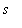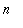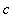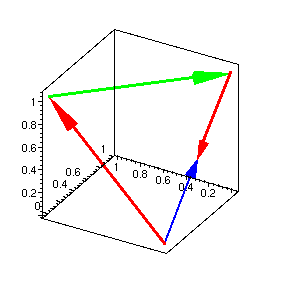Function: arrow3d - plot an arrow as a line with a pyramidal head.

Calling sequence:

arrow3d(base,vect);
Parameters:

base - base of the arrow, a 3D point ( list or vector)

vect - displacement (tip - base), a 3D point (list or vector)

Optional arguments:

scalefactor=to multiply length by real constant.

thickness=for thickness of the arrow stem (should be 0, 1, 2 or 3, default is 3).

colour=to specify arrow colour (default is red).

other 3D plot options

Description:

This function creates a 3D plot data object which, when displayed, is an arrow from base to base+vect (or base+s*vect if the option scalefactor=s is specified).

For an arrow from base to tip , use arrow3d(base,tip-base) .

Unless the option scaling=constrained ("Projection, Constrained" on the plot menu or "1-1" on the context bar) is used, the shape of the arrowhead may be distorted.

While it is mainly provided for use by the surfarrows and curvarrows functions, this function can be used on its own. Its result would usually be combined with other plot structures by the function display from the plots package.

This function is part of the package surfarro in the Maple Advisor Database library, and must be loaded before use by the command with(surfarro); or with(surfarro,arrow3d);

Examples:

> with(surfarro,arrow3d):

> a1:= arrow3d([0,0,0],[1,0,1]):
a2:= arrow3d([1,0,1],[0,1,1]-[1,0,1], colour=green):
a3:= arrow3d([0,1,1], vector([0,-1,-1]), scalefactor=1/2):
a4:= arrow3d([0,0,0],[0,1/2,1/2], colour=blue, thickness=2):
plots[display]({a1,a2,a3,a4},scaling=constrained, axes=box,orientation=[60,120]);